• 3.3 直方图处理 3.3.3 局部直方图处理 直方图均衡 和 直方图匹配 都是全局性的。虽然这种全局方法适用于整个图像的增强，但有时对图像小区域细节的局部增强也可以使用直方图处理。 局部增强 的解决方法就是在图像中...
第 3 章  灰度变换与空间滤波
3.3 直方图处理
3.3.3 局部直方图处理
直方图均衡 和 直方图匹配 都是全局性的。虽然这种全局方法适用于整个图像的增强，但有时对图像小区域细节的局部增强也可以使用直方图处理。
局部增强 的解决方法就是在图像中每一个像素的邻域中，根据灰度级分布（或其他特性）设置变换函数。
直方图处理技术很容易适应局部增强，该过程定义一个方形或矩形的邻域并把该区域的中心从一个像素移至另一个像素。在每一个位置的邻域中，该点的直方图都要被计算，并且得到的不是直方图均衡化就是规定化变换函数。这个函数最终将用来映射邻域中心像素的灰度。
示例 1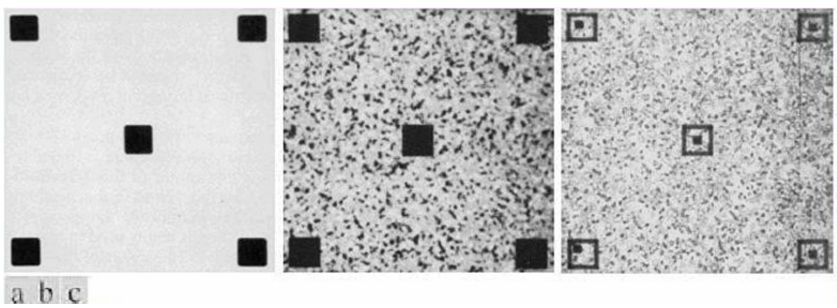图 (a) 显示了一幅被轻度模糊以减少噪声的图像。图 (b) 显示了全局直方图均衡的结果。当这种技术用于平滑噪声区域时，情况经常如此，对比度稍稍提高，噪声就会明显增强。可以说，这种方法没有带来新的结构性细节。然而，局部直方图均衡使用了一个 7*7 的邻域以显示在大的暗方形中的小方形。大小方形在灰度上很接近，但小方形的尺寸太小，故对全局直方图均衡化的影响不大。
示例 2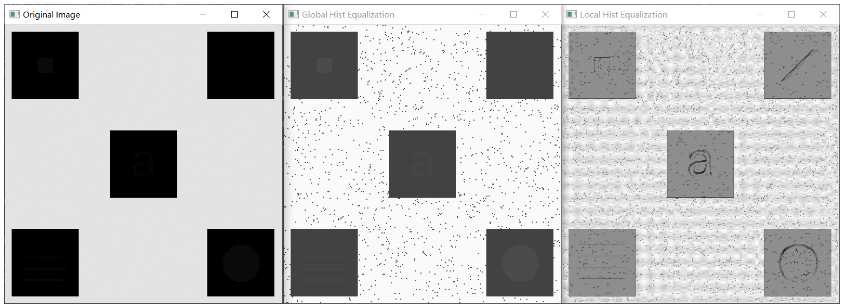如图，左图为原图像，中间图为全局直方图均衡的结果，右图为使用大小为 3*3 的邻域对左图应用局部直方图均衡的结果。
代码
#include <iostream>
#include <cmath>

#include <opencv2/core/core.hpp>
#include <opencv2/highgui/highgui.hpp>
#include <opencv2/imgcodecs/imgcodecs.hpp>
#include <opencv2/imgproc/imgproc.hpp>

void global_enhancement(cv::Mat& dst)
{
int Row = dst.rows;		int Col = dst.cols;
int grayScaleFrequence = { 0 };

for (int irow = 0; irow < Row; irow++)
for (int icol = 0; icol < Col; icol++)
grayScaleFrequence[dst.at<uchar>(irow, icol)]++;

for (int i = 0; i < 256; i++)
{
if (i < 255)
grayScaleFrequence[i + 1] += grayScaleFrequence[i];

grayScaleFrequence[i] = round((grayScaleFrequence[i] * 1.0) / (Row * Col) * 255);		// 全局直方图均衡映射表
}

for (int irow = 0; irow < Row; irow++)
for (int icol = 0; icol < Col; icol++)
dst.at<uchar>(irow, icol) = grayScaleFrequence[dst.at<uchar>(irow, icol)];

return;
}

{
int grayScaleFrequency = { 0 };

for (int irow = 0; irow < Row; irow++)
for (int icol = 0; icol < Col; icol++)

for (int i = 0; i < 256; i++)
{
if (i < 255)
grayScaleFrequency[i + 1] += grayScaleFrequency[i];

grayScaleFrequency[i] = round((grayScaleFrequency[i] * 1.0) / (Row * Col) * 255);		// 全局直方图均衡映射表
}

int result = grayScaleFrequency[mask.at<uchar>(Row / 2, Col / 2)];

return result;
}

void local_enhancement(cv::Mat& dst)
{
int length;
std::cout << "迭代邻域边长（默认邻域为正方形）：";
std::cin >> length;
int halfLength = length/2;

int Row = dst.rows;		int Col = dst.cols;
for (int irow = 0 + halfLength; irow < Row - halfLength; irow++)
{
for (int icol = 0 + halfLength; icol < Col - halfLength; icol++)
{
cv::Mat tempMask = dst(cv::Rect(icol - halfLength, irow - halfLength, length, length));
//cv::Mat tempMask = dst(cv::Rect(irow - halfLength, icol - halfLength, length, length));
}
}

return;
}

int main(int argc, char** argv)
{
cv::String imageName = "Fig0326.tif";

cv::Mat dst_global_enhancement = src.clone();
cv::Mat dst_local_enhancement = src.clone();
//cv::Mat dst_local_enhancement = src(cv::Rect(0, 40, 200, 50));

global_enhancement(dst_global_enhancement);
local_enhancement(dst_local_enhancement);

//for (int i = 10; i < 50; i++)
//	for (int j = 50; j < 90; j++)
//		dst_overall.at<uchar>(i, j) = 255;

cv::imshow("Original Image", src);
cv::imshow("Global Hist Equalization", dst_global_enhancement);
cv::imshow("Local Hist Equalization", dst_local_enhancement);

cv::waitKey(0);

return 0;
}

注意
cv::Rect rect(x, y, width, height);

在 OpenCV 中，坐标如图所示：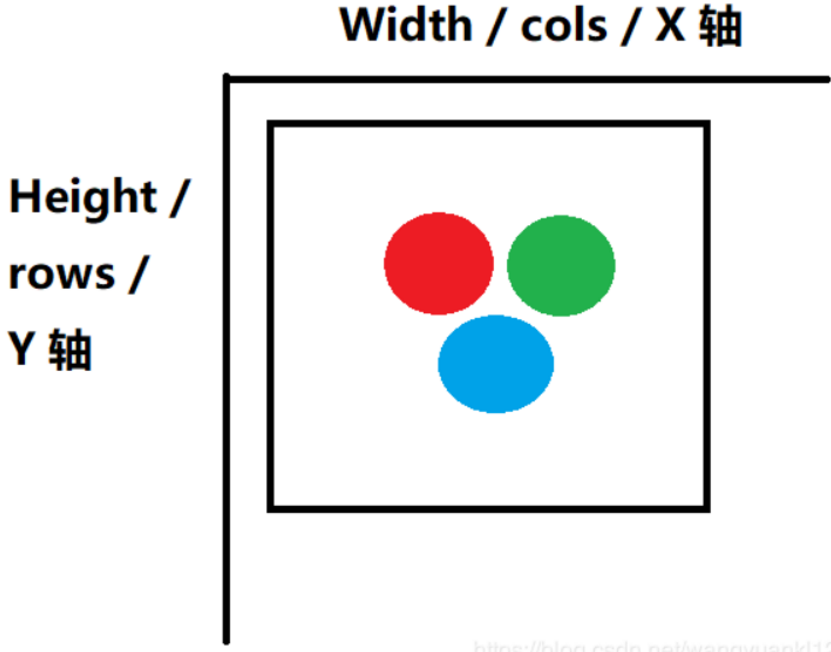在局部直方图均衡过程中，若程序写为：
cv::Mat tempMask = dst(cv::Rect(irow - halfLength, icol - halfLength, length, length));

意味着扫描图像时按照列进行扫描，则结果为：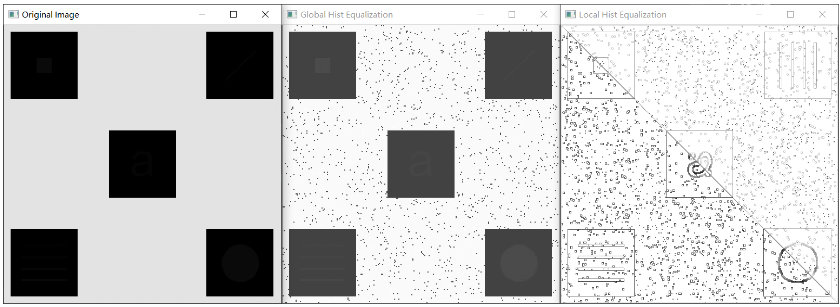展开全文opencv 数字图像处理
• 直方图处理直方图直方图显示参考代码效果图与应用场景直方图均衡参考代码效果图与应用场景直方图规定参考代码效果图与应用场景局部直方图均衡参考代码效果图与应用场景直方图统计参考代码效果图与应用场景 ...
直方图处理直方图直方图显示参考代码效果图与应用场景直方图均衡参考代码效果图与应用场景直方图规定参考代码效果图与应用场景局部直方图均衡参考代码效果图与应用场景直方图统计参考代码效果图与应用场景
直方图
直方图是图像像素灰度级的统计数目的直观表示。本文将从像素级操作完成直方图显示、直方图均衡、直方图规定（匹配）、直方图局部均衡以及直方图统计测试实验。
直方图显示
对于直方图的计算，我们需要统计各个灰度级上像素的数目，然后根据此关系勾画出直方图。直方图能够直观的显示图像像素在各个灰度级的分布，是其余实验的基础。
参考代码
#include<iostream>
#include<string>
#include<opencv2/core/core.hpp>
#include<opencv2/highgui/highgui.hpp>
#include<opencv2/opencv.hpp>
using namespace cv;
using namespace std;
void test1();
double* computeHist(Mat src);
void showHist(double* rank, string name);
double findNumber(double* num, int start, int end, int n);
void test2();
Mat myBalanceHist(Mat &src);
double* standHist(double* rank);
double* balanceHist(double* rank);
Mat mapping(Mat &src, double* rank);
void test3();
void standardImageSingleArray(Mat &src, double* num, int rank);
void gama_image(Mat &img, double gama, double rate);
Mat myMatchHist(Mat &src, double* dstHist);
void test4();
Mat myLocalBalance(Mat &src, int size);
void test5();
Mat statisticHist(Mat &src, double rate, double muk0, double sigmak1, double sigmak2, bool dark, int size);
Mat countMV(double* num, int nums);
int main()
{
test1();
test2();
test3();
test4();
test5();
return 0;
}

// 测试一
void test1()
{
// 读取灰度图像
// 显示直方图
double* rank = computeHist(img);
showHist(rank, "原图直方图");
imshow("原图", img);
waitKey(0);
destroyAllWindows();
}

// 计算直方图
double* computeHist(Mat src)
{
double* rank = new double;
// 初始化
for (int n = 0; n < 256; n++)
rank[n] = 0;
// 计算灰度分布
for (int i = 0; i < src.rows; i++)
for (int j = 0; j < src.cols; j++)
{
rank[src.at<uchar>(i, j)]++;
}
return rank;
}

// 显示直方图
void showHist(double* rank, string name)
{
Mat hist = Mat::zeros(255, 255, CV_8UC1);
// 深拷贝
double* rankCopy = new double;
for (int n = 0; n < 256; n++)
rankCopy[n] = rank[n];
double min = findNumber(rankCopy, 0, 256, 0);
double max = findNumber(rankCopy, 0, 256, 255);
for (int i = 0; i < 256; i++)
{
line(hist, Point(i, 255), Point(i, saturate_cast<int>(255 - (rank[i] - min) * 255 / (max - min))), Scalar(255));
}
imshow(name, hist);
}

// 寻找序列值
double findNumber(double* num, int start, int end, int n)
{
int p = start;
double stand = num[start];
for (int i = start + 1; i < end; i++)
{
if (num[i] < stand)
{
num[p] = num[i];
p++;
num[i] = num[p];
}
}
if (p == n)
return stand;
else if (p > n)
return findNumber(num, start, p, n);
else
return findNumber(num, p + 1, end, n);
}

效果图与应用场景直方图能够让观察者对图的灰度分布有一个直观的认识，方便后续图像增强工作的处理。

直方图均衡
直方图均衡是根据变换函数 $s = (L-1)\int_{0}^{r}w(r)\text{d}w$ 来进行映射的。原理大概可解释为在任意间隔内的灰度密度等于灰度间隔除以总灰度级，那么这个灰度分布就是均衡的。
$s+k = (L-1)\int_{0}^{r}w(r)\text{d}w+(L-1)\int_{r}^{r+t}w(r)\text{d}w$
$\frac{k}{L-1} =\int_{r}^{r+t}w(r)\text{d}w$
等式右边为灰度级从k到s+k所映射的像素占比为$\frac{k}{L-1}$，得证。
参考代码
// 测试二
void test2()
{
// 读取灰度图像
double* rank = computeHist(img);
Mat dst = myBalanceHist(img);
// 显示均衡后的直方图
double* rankBalance = computeHist(dst);
showHist(rank, "原图像直方图");
showHist(rankBalance, "均衡后的直方图");
imshow("原图", img);
imshow("直方图均衡", dst);
waitKey(0);
destroyAllWindows();
}

// 直方图均衡化
Mat myBalanceHist(Mat &src)
{
double* rank;
rank = computeHist(src);
// 归一化直方图
rank = standHist(rank);
// 直方图均衡
balanceHist(rank);
// 图像映射
Mat dst = mapping(src, rank);
return dst;
}

// 归一化直方图
double* standHist(double* rank)
{
// 深拷贝
double* rankCopy = new double;
for (int n = 0; n < 256; n++)
rankCopy[n] = rank[n];
double sum = 0;
for (int n = 0; n < 256; n++)
sum += rankCopy[n];
for (int n = 0; n < 256; n++)
rankCopy[n] /= sum;
return rankCopy;
}

// 直方图均衡
double* balanceHist(double* rank)
{
// 深拷贝
double* rankCopy = new double;
for (int n = 0; n < 256; n++)
rankCopy[n] = rank[n];
for (int i = 0; i < 256; i++)
{
for (int j = 0; j < i; j++)
rank[i] += rankCopy[j];
rank[i] *= 255;
}
return rank;
}

// 映射
Mat mapping(Mat &src, double* rank)
{
Mat dst = src.clone();
for (int i = 0; i < src.rows; i++)
for (int j = 0; j < src.cols; j++)
{
dst.at<uchar>(i, j) = saturate_cast<int>(rank[src.at<uchar>(i, j)]);
}
return dst;
}

效果图与应用场景对比原图与均衡后的图像，可发现变换图像的层次效果更加明显，直方图的分布基本占据整个灰> > 度级且各灰度级密度基本相当，而存在空缺的灰度级是因为图像灰度级是离散的，变换中存在多> > 个灰度级映射到同一个灰度级。

直方图均衡是自适应的，不需要对不同图像进行特定的调整，因此使用比较方便，应用场景较宽泛，多用于图像的预处理，增强图像的对比度。

直方图规定
直方图规定，或者称直方图匹配，算是直方图均衡的变种。直方图均衡化后图像的直方图是均匀分布的，而直方图规定化后图像的直方图分布是可控制的，因此需要提供一个各个灰度级的概率密度数据来进行匹配。
该算法的实现原理：

先对原图像进行直方均衡化，可得到一种映射关系$f(x)$；再对输入的灰度密度数据进行直方图均衡化，又可以得到一种映射关系$g(x)$。之后求$g(x)$的反函数$g^{-1}(x)$，可得到映射关系$f(g^{-1}(x))$，即原图像到目标概率密度的映射。但实际上灰度级是离散的，多个灰度级映射到一个灰度级上，$g(x)$可能是递增的但不是严格单调递增的，反函数可能不存在，这种情况需要进行一些特殊的处理，$g^{-1}(x)$如果输出对应多个则映射到最小值，如果输出不存在，则对应到差值最小的存在的灰度级上。

参考代码
// 测试三
void test3()
{
// 读取灰度图像
Mat dst = img.clone();
gama_image(dst, 2.5, 1);
// 绘制直方图
double* rankImg = computeHist(img);
double* rankDst = computeHist(dst);
// 直方图规定
Mat matchDst = myMatchHist(img, rankDst);
// 绘制规定的直方图
double* rankMatch = computeHist(matchDst);

showHist(rankImg, "原图直方图");
showHist(rankDst, "伽马直方图");
showHist(rankMatch, "规定直方图");
imshow("原图", img);
imshow("伽马变换", dst);
imshow("直方图规定", matchDst);
waitKey(0);
destroyAllWindows();
}

// 单通道图像标定——数组存储
void standardImageSingleArray(Mat &src, double* num, int rank = 255)
{
// 查找数组内的最大值与最小值
double min = 1000000000, max = -1000000000;
for (int i = 0; i < src.rows; i++)
for (int j = 0; j < src.cols; j++)
{
if (num[i*src.cols + j] < min)
min = num[i*src.cols + j];
if (num[i*src.cols + j] > max)
max = num[i*src.cols + j];
// cout << num[i*src.cols + j] <<endl;
}

// 对图像像素值进行标定
for (int i = 0; i < src.rows; i++)
for (int j = 0; j < src.cols; j++)
{
src.at<uchar>(i, j) = saturate_cast<int>(rank * ((num[i*src.cols + j] - min) / (max - min)));
}
}

void gama_image(Mat &img, double gama, double rate = 1)
{
double* num = new double[img.rows*img.cols];
int n = 0;
// 初始化图像迭代器
MatIterator_<uchar> srcIterStart = img.begin<uchar>();
MatIterator_<uchar> srcIterEnd = img.end<uchar>();

while (srcIterStart != srcIterEnd)
{
if (*srcIterStart == 0)
num[n] = 1.0;
else
num[n] = rate * pow(*srcIterStart, gama);
n++;
srcIterStart++;

}
standardImageSingleArray(img, num);

}

// 直方图规定化
Mat myMatchHist(Mat &src, double* dstHist)
{
// 均衡映射数组
double* srcRank = computeHist(src);
srcRank = standHist(srcRank);
srcRank = balanceHist(srcRank);
// 均衡规定映射数组
double* dstRank = standHist(dstHist);
dstRank = balanceHist(dstRank);

double* rank = new double;

for (int i = 0; i < 256; i++)
for (int j = 0; j < 256; j++)
{
if (srcRank[i] >= dstRank[j])
if (abs(srcRank[i] - dstRank[j]) <= abs(srcRank[i] - dstRank[j + 1]) || j == 255)
rank[i] = j;
}
Mat dst = src.clone();
dst = mapping(dst, rank);
return dst;
}

效果图与应用场景本测试， 使用伽马变换后的图像灰度分布当做规定模板，对比伽马变换图与规定直方图可知，两者区别不大，本次实验成功。直方图规定化可应用在指定灰度分布的作业上。

局部直方图均衡
全局直方图均衡是在整个图像中做一次均衡化， 而局部直方图均衡是在给定的模板大小的像素邻域内进行均衡化，有多少像素就得进行多少次均衡化，计算量较大。
参考代码
// 测试四
void test4()
{
// 读取灰度图像
Mat globalDst = myBalanceHist(img);
Mat localDst = myLocalBalance(img, 3);

imshow("原图", img);
imshow("直方图均衡", globalDst);
imshow("局部直方图均衡", localDst);
waitKey(0);
destroyAllWindows();
}

// 局部直方图均衡 ——待优化
Mat myLocalBalance(Mat &src, int size = 3)
{
Mat dst = src.clone();
for (int i = size / 2; i < (src.rows - size / 2); i++)
for (int j = size / 2; j < (src.cols - size / 2); j++)
{
// 初始化
double* num = new double;
for (int p = 0; p < 256; p++)
num[p] = 0;

for (int m = -size / 2; m <= size / 2; m++)
for (int n = -size / 2; n <= size / 2; n++)
{
num[dst.at<uchar>(i + m, j + n)]++;
}

num = standHist(num);
num = balanceHist(num);
dst.at<uchar>(i, j) = saturate_cast<int>(num[dst.at<uchar>(i, j)]);

delete[]num;
}
return dst;
}

效果图与应用场景局部直方图均衡可应用于显示局部对比度不强，而全局对比度又足够的图像，来获取局部的细节。

直方图统计
直方图统计也是根据模板大小内的像素邻域的均值、方差与全局的均值、方差的比较，来决定像素的操作。操作暗亮区域的图像只需要设置局部均值和全局均值的比值，而局部方差一般设置成小于全局的方差。
参考代码
// 测试五
void test5()
{
// 读取灰度图像
Mat darkDst = statisticHist(img, 10, 0.5, 0.02, 1, true, 3);
Mat lightDst = statisticHist(img, 0.1, 0.5, 0.02, 1, false, 3);
Mat globalDst = myBalanceHist(img);

imshow("原图", img);
imshow("直方图统计暗区域", darkDst);
imshow("直方图统计亮区域", lightDst);
imshow("直方图均衡", globalDst);
waitKey(0);
destroyAllWindows();
}

// 直方图统计
Mat statisticHist(Mat &src, double rate, double muk0, double sigmak1, double sigmak2, bool dark = true, int size = 3)
{
Mat dst = src.clone();
double mean, variance, lmean, lvariance;
double* num = new double[src.rows*src.cols];
double* lnum = new double[size*size];
int point;
for (int i = 0; i < src.rows; i++)
for (int j = 0; j < src.cols; j++)
num[i*src.rows + j] = src.at<uchar>(i, j);
Mat xy = countMV(num, src.rows*src.cols);
mean = xy.at<double>(0, 0);
variance = xy.at<double>(1, 0);
delete []num;

for (int i = size / 2; i < (src.rows - size / 2); i++)
for (int j = size / 2; j < (src.cols - size / 2); j++)
{
point = 0;
for (int m = -size / 2; m <= size / 2; m++)
for (int n = -size / 2; n <= size / 2; n++)
{
lnum[point] = dst.at<uchar>(i + m, j + n);
point++;
}
xy = countMV(lnum, size*size);
lmean = xy.at<double>(0, 0);
lvariance = xy.at<double>(1, 0);
if (dark)
{
if (lmean < muk0*mean && lvariance > sigmak1*variance && lvariance < sigmak2*variance)
dst.at<uchar>(i, j) = saturate_cast<int>(rate * dst.at<uchar>(i, j));

}
else
{
if (lmean > muk0*mean && lvariance > sigmak1*variance && lvariance < sigmak2*variance)
dst.at<uchar>(i, j) = saturate_cast<int>(rate * dst.at<uchar>(i, j));
}
}
return dst;
}

// 计算均值和方差
Mat countMV(double* num, int nums)
{
double sum = 0, mu, sigma;
for (int i = 0; i < nums; i++)
{
sum += num[i];
}
mu = sum / nums;

sum = 0;

for (int i = 0; i < nums; i++)
{
sum += pow(num[i] - mu, 2);
}
sigma = sum / nums;
Mat xy = (Mat_<double>(2, 1) << mu, sigma);
return xy;
}

效果图与应用场景直方图统计可指定灰度且对比差的区域进行相应的提升。


展开全文cpp opencv 图像增强
• python 图像直方图处理
python 图像直方图处理

预备知识

reshape函数

vec=np.arange(15)
print vec
结果：[ 0  1  2  3  4  5  6  7  8  9 10 11 12 13 14]

mat= vec.reshape(3,5)
print mat
结果：
[[ 0  1  2  3  4]
[ 5  6  7  8  9]
[10 11 12 13 14]]

现在如果我们返过来，知道一个二维矩阵，要变成一个一维数组，就不能用reshape了，只能用flatten.

flatten函数

a1=mat.reshape(1,-1)  #-1表示为任意，让系统自动计算
print a1
a2=mat.flatten()
print a2
a1:  [[ 0  1  2  3  4  5  6  7  8  9 10 11 12 13 14]]
a2:  [ 0  1  2  3  4  5  6  7  8  9 10 11 12 13 14]

综上：
可以看出，用reshape进行变换，实际上变换后还是二维数组，两个方括号，因此只能用flatten.我们要对图像求直方图，就需要先把图像矩阵进行flatten操作，使之变为一维数组，然后再进行统计。

灰度图像

#

绘图都可以调用matplotlib.pyplot库来进行，其中的hist函数可以直接绘制直方图。
调用方式：

n, bins, patches = plt.hist(arr, bins=50, normed=1, facecolor='green', alpha=0.75)


hist的参数
常用的就这五个，只有第一个是必须的，后面四个可选

arr: 需要计算直方图的一维数组
bins: 直方图的柱数，可选项，默认为10
normed: 是否将得到的直方图向量归一化。默认为0（不归一化）
facecolor: 直方图颜色
alpha: 透明度
返回值 ：

n: 直方图向量，是否归一化由参数设定
bins: 返回各个bin的区间范围
patches: 返回每个bin里面包含的数据，是一个list
核心代码：


gray = np.array(img).flatten()
n, bins, patches = plt.hist(arr, bins=256, normed=1, facecolor='green', alpha=0.75)  

直方图展示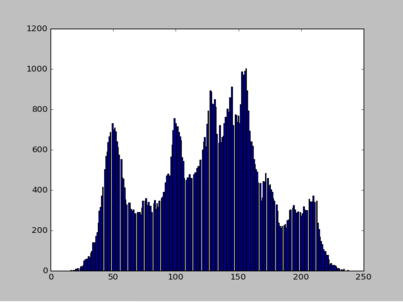彩色图像

核心代码

img = cv2.imread(imgfile,3)
r,g,b=src.split()  # 将rbg三通道分开
plt.figure("lena")
ar=np.array(r).flatten()
n,bins,patches=plt.hist(ar,bins=256,normed=1,facecolor='r',edgecolor='r',hold=1)
# n:归一化后的每个灰度值的累计数值shape为256  bin：直方图中柱状的数目
ag=np.array(g).flatten()
plt.hist(ag,bins=256,normed=1,facecolor='g',edgecolor='g',hold=1)
ab=np.array(b).flatten()
plt.hist(ab,bins=256,normed=1,facecolor='b',edgecolor='b')
plt.show()     

直方图效果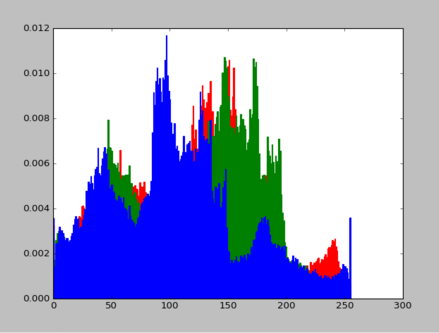展开全文python
• ## 二、数字图像处理之直方图处理

千次阅读 多人点赞 2019-04-03 21:15:05
数字图像处理之直方图处理直方图处理概念直方图均衡化（Histogram Equalization）直方图规定化局部直方图处理 直方图处理概念 灰度级范围为[0,L-1]的数字图像的直方图是离散函数h（rk）=nk，其中rk是第k级灰度值，nk...
数字图像处理之直方图处理直方图处理概念直方图均衡化（Histogram Equalization）直方图规定化（Histogram Specification）局部直方图处理
直方图处理概念
灰度级范围为[0,L-1]的数字图像的直方图是离散函数h（rk）=nk，其中rk是第k级灰度值，nk是图像中灰度为rk的像素个数。在实践中，经常用乘积MN表示的图像像素的总数除它的每个分量来归一化直方图，通常M和N是图像的行和列的维数。因此，归一化后的直方图由p（rk）=nk/MN给出，其中k=0,1,…,L-1。简单来说，p（rk）是灰度级rk在图像中出现的概率的一个估计。归一化直方图的所有分量之和应等于1。
在直方图中，暗图像集中分布在灰度级的低端；亮图像集中分布在灰度级的高端。低对比度图像具有较窄的直方图，且集中于灰度级的中部；高对比度图像的直方图分量则覆盖了很宽的灰度级范围。
直方图均衡化（Histogram Equalization）
均衡化的基本原理：是把原始图的直方图变换为均匀分布的形式，这样就增加了象素灰度值的动态范围从而可达到增强图像整体对比度的效果。
均衡化步骤：

统计图像中每个灰度级出现的次数，计算图像中每个灰度级出现的概率；
根据变换公式得到直方图均衡化的变换函数；
根据变换函数映射到每个像素点；
输出映射后的图像；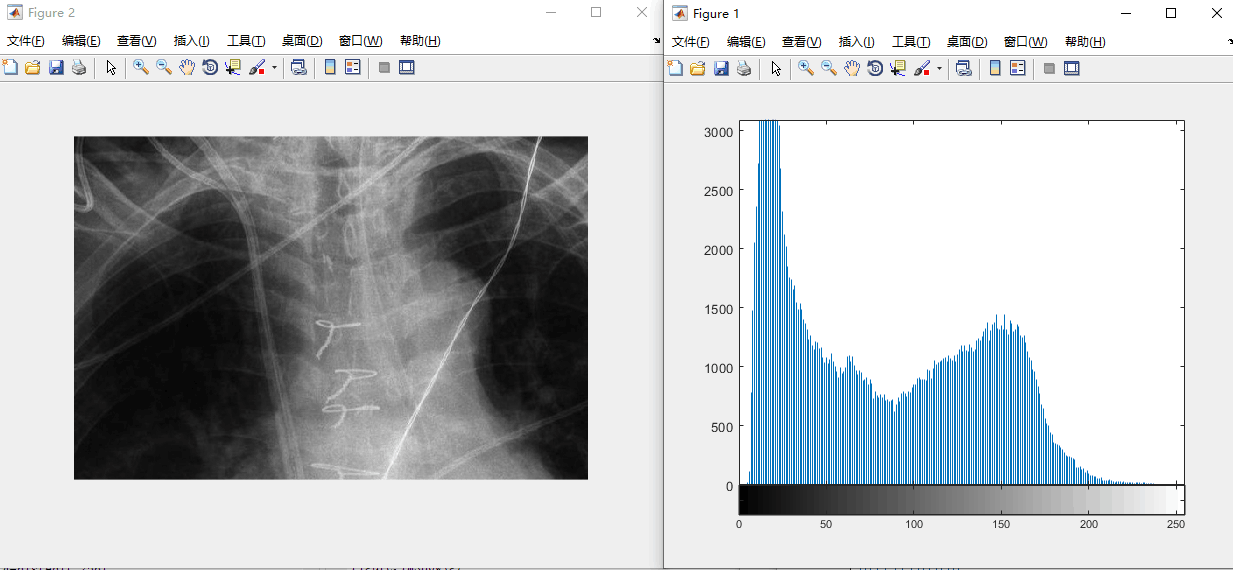均衡化之前的图像

clear all
>> f=rgb2gray(f);
>> imshow(f);
>> imhist(f);
figure,imshow(f);
g=histeq(f,256);
figure,imhist(g);
figure,imshow(g);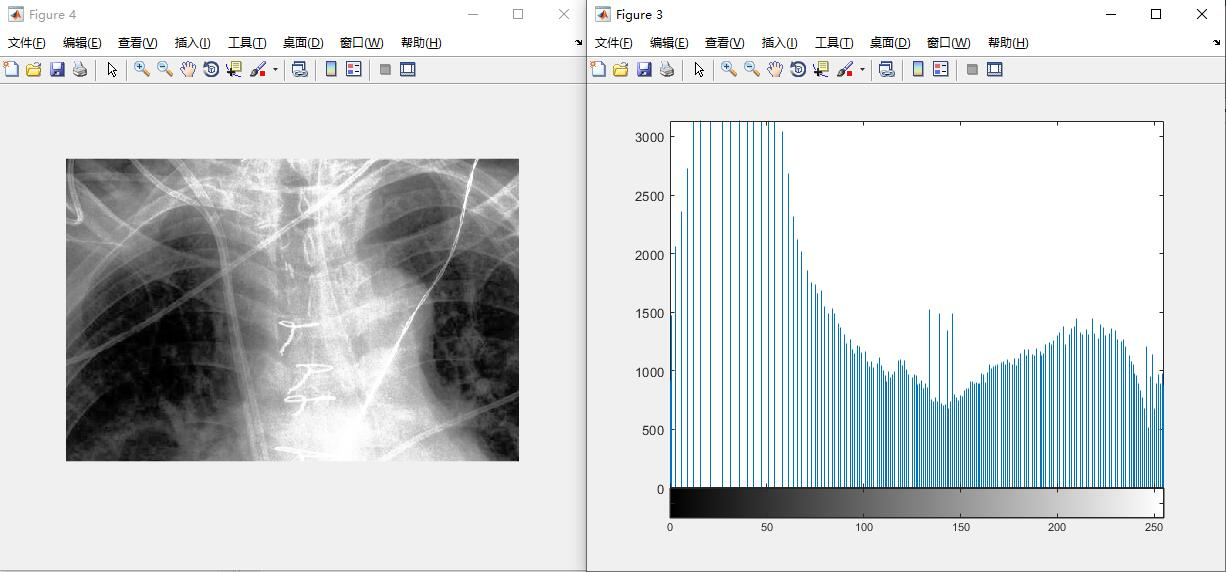均衡化之后的图像
直方图规定化（Histogram Specification）
规定化的基本原理：有目的的增强某个灰度区间的图像，即能够人为地修正直方图的形状，使之与期望的图像相匹配。
规定化步骤：
计算给定图像的直方图pr®，并用它寻找直方图均衡变化，把sk四舍五入为范围[0，L-1]内的整数；
计算变化函数G的所有值，把变换函数G的值四舍五入为范围[0，L-1]内的整数，并将G的值放入一个表中；
对每一个值sk，使用步骤2存储的G值寻找相应的zq值，以使G（zq）最接近sk，并存储这些从s到z的映射。当满足给定sk的zq值多余一个时（即映射不唯一时），则选择最小的值；
首先对输入图像进行均衡，然后用步骤3找到的映射把该图像中的每个均衡后的像素值sk映射为直方图规定化后的图像中的相应zq的值，形成直方图规定化后的图像。
clear all
r=127;
x=-r:r+1;
sigma=20;
y1=exp(-((x-80).^2)/(2*sigma^2));
y2=exp(-((x+80).^2)/(2*sigma^2));
y=y1+y2;                        %双峰高斯函数，任意函数都可以

y=y/sum(y);         %归一化，使函数符合概率分布的sum(y)==1这样一个规律
plot(y);            %待匹配的直方图

G=[];               %函数的累积直方图
for i=1:256
G=[G sum(y(1:i))];
end

img=rgb2gray(img);
[m n]=size(img);
hist=imhist(img);       %待处理图像的直方图
p=hist/(m*n);
figure;plot(p)          %原图直方图

s=[];                   %待处理图像的累积直方图
for i=1:256
s=[s sum(p(1:i))];
end

for i=1:256
tmp{i}=G-s(i);
tmp{i}=abs(tmp{i});         %因为要找距离最近的点，所以取绝对值
[a index(i)]=min(tmp{i});   %找到两个累积直方图距离最近的点
end

imgn=zeros(m,n);
for i=1:m
for j=1:n
imgn(i,j)=index(img(i,j)+1)-1;    %由原图的灰度通过索引映射到新的灰度
end
end

imgn=uint8(imgn);
figure;imshow(imgn)
figure;plot(imhist(imgn))       %新图的直方图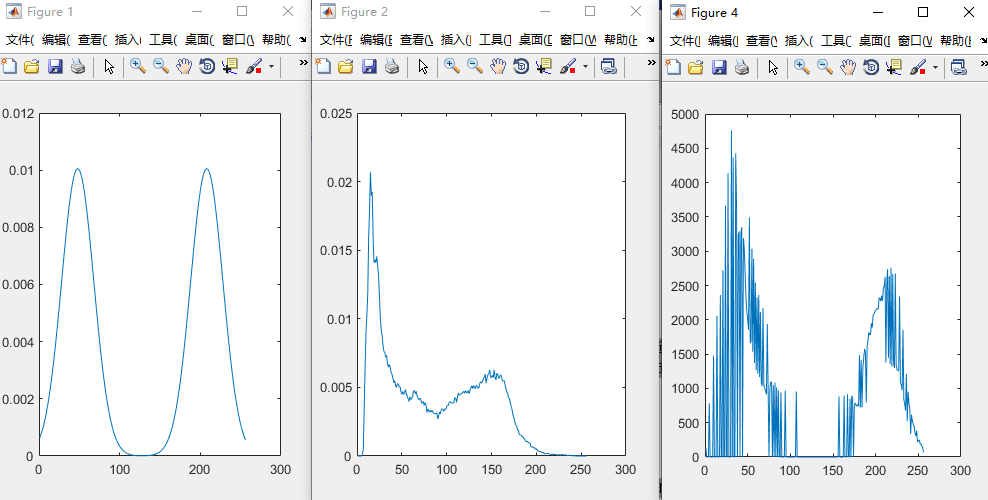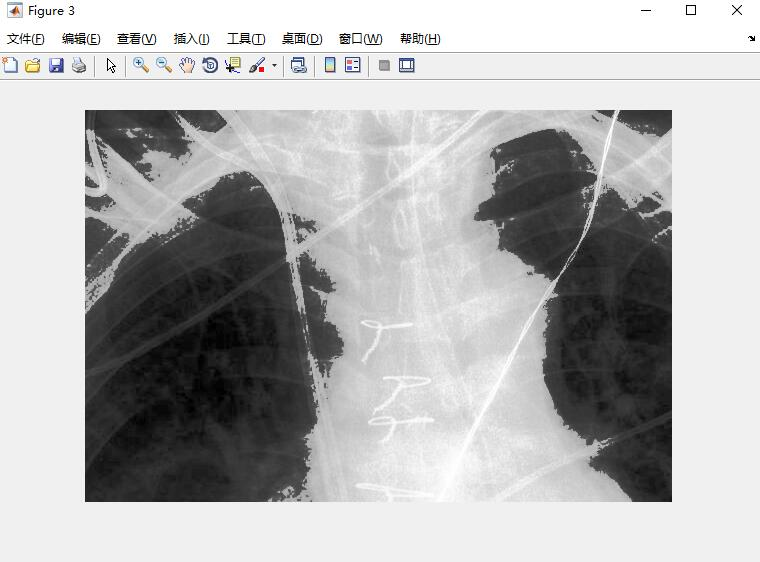局部直方图处理
在某种意义上，像素被基于整幅图像的灰度分布的变换函数修改。虽然这种全局方法适用于整个图像的增强，但存在这样的情况，增强图像中小区域的细节也是需要的。这些区域中，一些像素的影响在全局变换的计算中可能被忽略了，因为全局变换没有必要保证期望的局部增强。解决方法是以图像中每个像素的邻域中的灰度分布为基础设计变换函数。
前面描述的直方图处理技术很容易适应局部增强。该过程是定义一个邻域，并把该区域的中心从一个像素移至另一个像素。在每一个位置，计算邻域中的点的直方图，并且得到的不是直方图均衡化，就是规定化变换函数。这个函数最终用于映射邻域中心像素的灰度。然后，邻域中心的被移至一个相邻像素位置，并重复该过程。当邻域进行逐像素平移时，由于只有邻域中的一行或一列改变，所以可在每一步移动中，以新数据更新前一个位置得到的直方图。这种方法与区域每移动一个像素位置就计算邻域中所有像素的直方图相比有明显的优点。有时用于减少计算量的另一种方法是使用非重叠区域，但这种方法通常会产生我们不希望的“棋盘”效应。


展开全文• 图像处理冈萨雷斯局部直方图处理(Local Histogram Processing)局部直方图均衡化解决办法：直方图统计(Histogram Statistics)合理的创建标题，有助于目录的生成如何改变文本的样式插入链接与图片如何插入一段漂亮的...计算机视觉
• 直方图处理 直方图是图像处理过程中的一种非常重要的分析工具。直方图从图像内部灰度级的角度对图像进行表述，包含十分丰富而重要的信息。从直方图的角度对图像进行处理，可以达到增强图像显示效果的目的。 1、 直方...
• 3.3 直方图处理 3.3.2 直方图匹配（规定化） 直方图均衡能自动地确定变换函数，该函数寻求产生有均匀直方图的输出图像，需要自动增强时，这是一个好方法。 但是在某些情况下，采用均匀直方图的基本增强并不是最好的...数字图像处理 直方图匹配 规定化
• 如果我们要谈论局部直方图处理，那么不得不提起与之相对应的全局直方图处理，之前写过一篇文章关于全局直方图处理的，对全局直方图不熟悉的话可以先去看一下，这样再看局部直方图处理会更好。 当我想让一幅图想的...opencv 计算机视觉 算法 c++
• 直方图均衡生成的是自适应的变换函数，是以图像的直方图为基础。但是一旦一幅图像的变换函数计算完毕，它将不再改动，除非直方图有变动。 生成具有指定直方图的图像的方法称为直方图匹配或直方图规定化 文章目录一...《数字图像处理》
• 数字图像处理数字图像处理---灰度变换与空间滤波（一）直方图处理与函数绘图1.1生成并绘制图像的直方图（二）在频率域中直接生成滤波器（三）高通（锐化）频域滤波器（四）选择性滤波（五）小结 数字图像处理—灰度...
• 3.3 直方图处理   直方图是多种空间域处理技术的基础。   灰度级范围为 [0, L-1] 的数字图像的直方图是离散函数 h(rk)=nk h(r_k) = n_k h(rk​)=nk​ 这里 rk 是第 k 级灰度，nk 是图像中灰度级为 rk 的像素个数...数字图像处理 直方图均衡
• 欢迎关注“小白玩转Python”，发现更多 “有趣”引言在昨天的文章中我们介绍了基于灰度图像的直方图处理，也简单的提到了彩色图像的直方图处理，但是没有讨论最好的方法。让我们从导入所有需...列表 python 计算机视觉 opencv 微软
• 循序渐进之（二）空间域图像增强之直方图处理直方图均衡化(HE) 冈萨雷斯第二版《数字图像处理》，直方图均衡化是3.3.1章节的内容。 （拓展： HE算法：直方图均衡化。。 AHE算法：自适应直方图均衡化。。 ...直方图均衡化 图像增强
• 处理直方图的工具箱中，核心函数imhist：h = imhist(f,b) 式中，f为输入图像，h为其直方图，b是用来形成直方图的“容器”的数目（若b未包含在此参量中，则其默认值为256） 一个容器仅是灰度范围的一小部分， 例如...《数字图像处理》
• 直方图均衡由函数histeq实现：g = histeq(f,nlev) 其中，f 为输入图像，nlev是为输出图像指定的灰度级数。若nlev等于L(输入图像中可能的灰度级的总数)，则histeq直接执行变换函数T(rk)；若nlev小于L，则histeq...直方图均衡
• 文章目录直方图处理直方图的均衡化直方图的规定化 直方图处理 灰度直方图是灰度级的函数，描述的是图像中具有该灰度级的像元的个数。以横轴表示灰度级，以纵轴表示每一灰度级具有的像元数或该像元数占总像元数的比例...
• 1.直方图处理概念 灰度级范围为[0,L-1]的数字图像的直方图是离散函数h（rk）=nk，其中rk是第k级灰度值，nk是图像中灰度为rk的像素个数。在实践中，经常用乘积MN表示的图像像素的总数除它的每个分量来归一化直方图，...
• 文章目录前言一、直方图均衡1.处理方法二、使用步骤1.引入库2.读入数据总结 前言 直方图反映了图像整体灰度级的分布情况。 一、直方图均衡 顾名思义，人为使图像的灰度级分布更加均衡。 1.处理方法 二、使用步骤 1...计算机视觉 matlab
• 图像的直方图图像的直方图处理是从概率统计的角度出发，对图像灰度级的概率分布进行变换，从而达到使图像细节丰富、动态范围较大、便于观测和理解的目的。直方图处理是多种空间域处理技术的基础，可以直接用于图像的...冈萨雷斯数字图像处理 数字图像基础 Matlab
• 局部直方图处理是弄一个略大于图片的矩阵，超过图片的部分用0来代替像素值，在这个局部进行直方图均衡化。 输入： import cv2 import numpy as np import matplotlib.pyplot as plt import datetime # 局部直方图...
• 灰度级范围为[0,L-1]的数字图像的直方图是离散函数： 其中是第k级灰度值(=k)，是图像中灰度值为的像素个数。 通常用MN表示的图像像素的总数除它的每个分量来归一化直方图，即： M和N分别是图像的行和列维数，...图像处理 直方图均衡 直方图规定化
• 比如直方图处理，直方图常见的有直方图均衡化，规定化或者其他变换，一般是针对整幅图像而言。其实这些方法一样适用于局部图像增强，一般以像素的某个邻域作为处理范围。同样，局部处理的算法也可以扩展到全局算法。...算法 扩展
• 灰度直方图灰度直方图是灰度级的函数，描述的是图像中具有该灰度级的像元的个数。确定图像像元的灰度值范围，以适当的灰度间隔为单位将其划分为若干等级，以横轴表示灰度级，以纵轴表示每一灰度级具有的像元数或该像...数字图像处理
• 2.3 直方图处理与函数绘图 以从图像灰度直方图中提取的信息为基础的灰度变换函数，在诸如增强、压缩、分割、描述等方面的图像处理中起着重要作用。 一幅数字图像在[0,G]范围内共有L个灰度级，其直方图定义为下列...
• 直方图通常是用于图像增强，例如直方图均衡即是对图像对比度做调整处理直方图均衡的基本思想是把原始图的直方图变换为均匀分布的形式，这样就增加了像素灰度值得动态范围从而可达到增强图像整体效果的目的 映射...直方图均衡
• 对数及对比度拉伸变换、直方图处理与函数绘图。 对数及对比度拉伸变换 对数及对比度拉伸变换的作用主要是可以增强图片里较暗部分的细节，公式如下所示 c⋅logv+1(1+v⋅r)rϵ[0,1] c \cdot log_{v+1}^{(1+v \cdot...matlab
• 直方图处理4.1 生成并绘制图像直方图4.2 直方图均衡化 空间域图像增强 图像增强是图像处理中最具吸引力的领域之一，增强处理的首要目标是处理图像，对图像进行加工，使其比原始图像更适合特定的应用。空间域是指...计算机视觉
• ## 灰度直方图处理

千次阅读 2018-05-31 01:11:07
（一）直方图均衡 我们注意到，在暗的图像中，其直方图的分量主要集中在灰度级较暗的一段。同样，低对比度的图像，其直方图的分量较窄。可以设想，若将直方图的分量均匀地平铺在灰度级上，可以得到一幅灰度细节丰富...
• 直方图规定化的基本思想：有目的的增强某个灰度区间的图像，即能够人为地修正直方图的形状，使之与期望的图像相匹配。 直方图规定化是在运用均衡化原理的基础上，通过建立原始图像和期望图像之间的关系，选择地...直方图匹配
• ## 《数字图像处理》--直方图处理

万次阅读 多人点赞 2016-10-08 17:33:23
直方图灰度直方图是灰度级的函数，描述的是图像中具有该灰度级的像元的个数。确定图像像元的灰度值范围，以适当的灰度间隔为单位将其划分为若干等级，以横轴表示灰度级，以纵轴表示每一灰度级具有的像元数或该像元数...数字图像处理...